Science Courses / Course / Chapter

# What is a Pendulum in Physics?

Katherine Kaylegian-Starkey, David Wood, Amanda Robb
• Author
Katherine Kaylegian-Starkey

Katherine has a bachelor's degree in physics, and she is pursuing a master's degree in applied physics. She currently teaches struggling STEM students at Lane Community College.

• Instructor
David Wood

David has taught Honors Physics, AP Physics, IB Physics and general science courses. He has a Masters in Education, and a Bachelors in Physics.

• Expert Contributor
Amanda Robb

Amanda has taught high school science for over 10 years. She has a Master's Degree in Cellular and Molecular Physiology from Tufts Medical School and a Master's of Teaching from Simmons College. She is also certified in secondary special education, biology, and physics in Massachusetts.

Understand the definition of a pendulum in physics. Learn how Newtonian mechanics describes the motion of pendulums, their period and frequency, through equations. Updated: 10/17/2021

Show

## Pendulum Definition in Physics

A pendulum is defined as a free-swinging mass anchored to a fixed point. In physics, when studying pendulums, it is customary to model the motion using a simple pendulum. A simple pendulum is a simplified model that assumes that the tether is massless and the mass is a spherical and uniform point-mass. There are two defining characteristics of a pendulum besides its physical makeup: simple harmonic motion and a dependence upon gravity. Simple harmonic motion, or SHM, is an oscillatory motion created by a restoring force around an equilibrium point, and the motion of a pendulum is only one form of SHM. Gravity is also a defining characteristic of a pendulum because, even though some pendulums have an external driving force, it is gravity trying to pull the mass back to equilibrium that creates the characteristic oscillatory motion of a pendulum.

### Simple Harmonic Motion in Pendulum Physics

The concepts of "oscillatory motion" and "equilibrium point" are crucial to understanding pendulums.

Oscillatory motion: Movement that predictably repeats. It is often modeled mathematically by sine waves.

Equilibrium point: A place within a system where the system is balanced. Some equilibrium points are stable, and it is difficult for the system to leave this point. Other equilibrium points are unstable, and the system changes readily.

When a pendulum swings its mass sweeps out an arc. The point that is closest to the ground typically is the center of the arc, and this is the pendulum's equilibrium point. It isn't stable, however, so as the pendulum swings it easily passes equilibrium. Gravity pulls on the mass, and it once again sweeps back through the arc as it tries to stay as close to the ground a possible. This is why a dependence on gravity is a defining characteristic of a pendulum. With each successive sweep across equilibrium, the pendulum's kinetic energy turns into potential energy, and the sweeps have lower and lower amplitudes. The amplitude of a wave is how far above 0 it extends. If the pendulum is driven, then each successive sweep will have the same kinetic energy as before so the amplitude of the arc will remain constant. It is this repeated sweep through equilibrium that makes pendulum motion simple harmonic motion.

## What Is a Pendulum?

A pendulum is a weight hung from a stationary point in a way that allows it to swing freely back and forth. A simple pendulum is one where the pendulum bob is treated as a point mass, and the string from which it hangs is of negligible mass. Simple pendulums are interesting from a physics perspective because they are an example of simple harmonic motion, much like springs or rubber bands can be.

Simple harmonic motion is any periodic motion where a restoring force is applied that is proportional to the displacement and in the opposite direction of that displacement. Or in other words, the more you pull it one way, the more it wants to return to the middle. This is easy to imagine with a spring because you feel the increased tug as you stretch it more and more.

But what about a pendulum? Well, when you lift a pendulum to one side, the force of gravity wants to pull it back down, and the tension in the string wants to pull it left (or right). These combined forces work together to pull it back towards the middle (the equilibrium position). Ultimately, upon reaching the middle, the pendulum's velocity has increased, so it continues past the equilibrium position and off to the other side. This pattern then continues.

With a pendulum (or any simple harmonic motion), the velocity is greatest in the middle, but the restoring force (and therefore the acceleration) is greatest at the outer edges.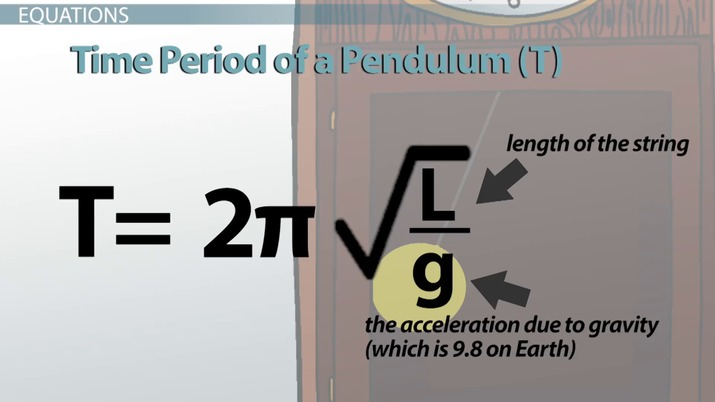An error occurred trying to load this video.

Try refreshing the page, or contact customer support.

Coming up next: Differences Between Translational & Rotational Motion

### You're on a roll. Keep up the good work!

Replay
Your next lesson will play in 10 seconds
• 0:01 What is a Pendulum?
• 1:30 Equations
• 2:55 Example Problem
• 4:26 Lesson Summary
Save Save

Want to watch this again later?

Timeline
Autoplay
Autoplay
Speed Speed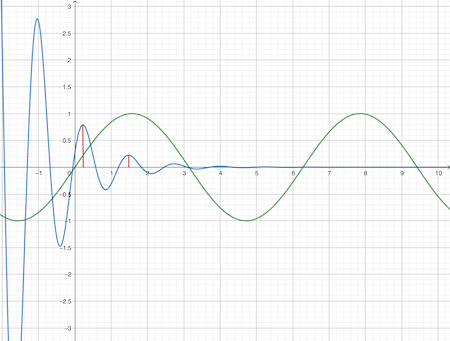## Pendulum Equation

The SHM of a pendulum can be described using Newtonian mechanics. Newtonian mechanics relate force to mass and acceleration with the equation F = ma. For a simple pendulum the only force acting on it is gravity so a = -g, assuming that +y is up in the coordinate system. Because the motion of the pendulum makes an arc gravity cannot be just g. The mass will feel gravity differently depending on its position along the arc, and the force equation needs to represent this. This final piece is incorporated by viewing the whole system as a triangle and using {eq}sin (\theta) {/eq} so that {eq}F = -mgsin( \theta) {/eq}.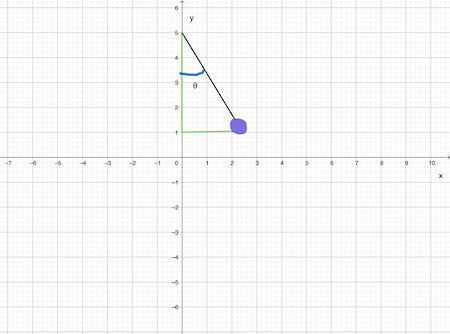In practice finding {eq}\theta {/eq} is often impossible, but the angle the mass travels through is the same its angular frequency times time, {eq}\omega t {/eq}. Frequency is how many oscillations occur in a given time, and angular frequency relates that time to the time it would take for the mass to travel a full circle. Angular frequency is {eq}\omega = \sqrt(g/L) {/eq}, and frequency is {eq}(1/2\pi) \sqrt(g/L) {/eq} where L is the distance the mass is from the pivot point.

### What is Meant by the Period of a Pendulum?

It is next to impossible to talk about frequency without talking about period. The period of a pendulum is how long it takes the pendulum to make a pass through the equilibrium point and return again. Mathematically it is the reciprocal of frequency, {eq}T = 2\pi \sqrt(L/g) {/eq}. T is the conventional way to represent period, and L, like in the previous equations, is the distance the mass is from the pivot point. A factor of {eq}2\pi {/eq} appears because a typical sinusoidal oscillation has a period of {eq}2\pi {/eq} and all oscillations are a transformation of a basic sine wave.

## Example of Pendulum Computations

Let's do three examples to use the equations introduced above.

## Equations

There are many equations to describe a pendulum. One equation tells us that the time period of the pendulum, T, is equal to 2pi times the square-root of L over g, where L is the length of the string, and g is the acceleration due to gravity (which is 9.8 on Earth).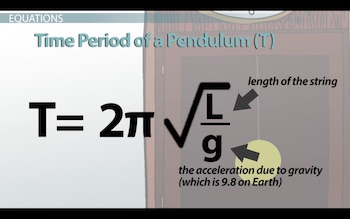But since all simple harmonic motion is sinusoidal, we also have a sine equation: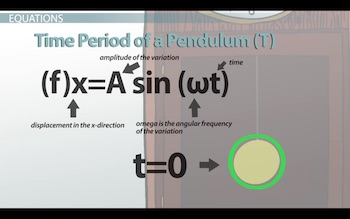This one says that the displacement in the x-direction is equal to the amplitude of the variation, A (otherwise known as the maximum displacement), multiplied by sine omega-t, where omega is the angular frequency of the variation, and t is the time. In this equation, you start your mathematical stopwatch in the middle - time, t=0, is right in the middle as it swings by.

Finally, you might be wondering: what is angular frequency? Well, angular frequency is the number of radians that are completed each second. A full 360 degrees is 2pi radians, and that represents one full oscillation: from the middle to one side, back to the middle to the other side, and then back to the middle again. You can convert this angular frequency to regular frequency by dividing the angular frequency by 2pi. Regular frequency just tells you the number of complete cycles per second and is measured in hertz.

## Example Problem

Okay, let's go through an example. A pendulum that is 4 meters in length completes one full cycle 0.25 times every second. The maximum displacement the pendulum bob reaches is 0.1 meters from the center. What is the time period of the oscillation? And what is the displacement after 0.6 seconds?

To unlock this lesson you must be a Study.com Member.

Video Transcript

## What Is a Pendulum?

A pendulum is a weight hung from a stationary point in a way that allows it to swing freely back and forth. A simple pendulum is one where the pendulum bob is treated as a point mass, and the string from which it hangs is of negligible mass. Simple pendulums are interesting from a physics perspective because they are an example of simple harmonic motion, much like springs or rubber bands can be.

Simple harmonic motion is any periodic motion where a restoring force is applied that is proportional to the displacement and in the opposite direction of that displacement. Or in other words, the more you pull it one way, the more it wants to return to the middle. This is easy to imagine with a spring because you feel the increased tug as you stretch it more and more.

But what about a pendulum? Well, when you lift a pendulum to one side, the force of gravity wants to pull it back down, and the tension in the string wants to pull it left (or right). These combined forces work together to pull it back towards the middle (the equilibrium position). Ultimately, upon reaching the middle, the pendulum's velocity has increased, so it continues past the equilibrium position and off to the other side. This pattern then continues.

With a pendulum (or any simple harmonic motion), the velocity is greatest in the middle, but the restoring force (and therefore the acceleration) is greatest at the outer edges.

## Equations

There are many equations to describe a pendulum. One equation tells us that the time period of the pendulum, T, is equal to 2pi times the square-root of L over g, where L is the length of the string, and g is the acceleration due to gravity (which is 9.8 on Earth).But since all simple harmonic motion is sinusoidal, we also have a sine equation:This one says that the displacement in the x-direction is equal to the amplitude of the variation, A (otherwise known as the maximum displacement), multiplied by sine omega-t, where omega is the angular frequency of the variation, and t is the time. In this equation, you start your mathematical stopwatch in the middle - time, t=0, is right in the middle as it swings by.

Finally, you might be wondering: what is angular frequency? Well, angular frequency is the number of radians that are completed each second. A full 360 degrees is 2pi radians, and that represents one full oscillation: from the middle to one side, back to the middle to the other side, and then back to the middle again. You can convert this angular frequency to regular frequency by dividing the angular frequency by 2pi. Regular frequency just tells you the number of complete cycles per second and is measured in hertz.

## Example Problem

Okay, let's go through an example. A pendulum that is 4 meters in length completes one full cycle 0.25 times every second. The maximum displacement the pendulum bob reaches is 0.1 meters from the center. What is the time period of the oscillation? And what is the displacement after 0.6 seconds?

To unlock this lesson you must be a Study.com Member.

In this activity, students are going to build their own pendulum and calculate the period using the equation for the lesson and compare it to an actual measurement from observation of the pendulum. To do this activity you'll need a ring stand, a C-clamp, about 20cm of string and a washer.

### Student Instructions

Now that you're familiar with what a pendulum is and some of the equations, it's time to build your own. Follow the instructions below to build a pendulum, then we'll calculate and observe the period and compare our results.

1. Attach the C-clamp to the ring stand so that it is perpendicular to the stand.
2. Cut a string that is 0.2m long, or just long enough so that there is an inch between the ground and the string when it is tied. Tie the string to the C-clamp.
3. Next, tie a washer to the bottom of the string.
4. Measure the distance between the top of the string and the bottom of the washer. This is the length (L) of the pendulum.
5. Use the equation in the lesson to calculate the period. Record your work and your final answer.
6. Next, raise the pendulum and let it go. Record how long it takes the pendulum to complete one back and forth motion in seconds. This is the observed period.
7. Next, Repeat this trial four times and take the average observed period.

### Questions

1. How did the calculated period compare to the observed period? If there was a difference explain why you think that could have happened.
2. How did the average observed period differ from the single observation? Why do we take an average of values in science?
3. Explain how this experiment demonstrated simple harmonic motion.
4. What are some applications for studying the period of a pendulum?

### Expected Results

Students should see a slight discrepancy in the actual versus observed period. The observed period may be longer because of interaction with air resistance and friction. The accuracy of students' measurements can also cause a discrepancy between calculated and observed results. The pendulum shows simple harmonic motion because as the pendulum is pulled one way, the motion when released is equal but opposite. Over time the pendulum slows down due to air resistance.

### Register to view this lesson

Are you a student or a teacher?

Back

### Resources created by teachers for teachers

Over 30,000 video lessons & teaching resources‐all in one place.Video lessonsQuizzes & WorksheetsClassroom IntegrationLesson Plans

I would definitely recommend Study.com to my colleagues. It’s like a teacher waved a magic wand and did the work for me. I feel like it’s a lifeline.

Jennifer B.
TeacherCreate an account to start this course today
Used by over 30 million students worldwide